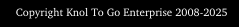Physics Formulae
Transformers

P = VI or VI = P

where
P =
power
V = voltage
I = current

This is an appropriate formula to use to determine the current of the a.c. input supply, given the power and voltage of the source.

============
current, I = P/V
============

The e.m.f. or voltage induced in a coil is dependent on the number of turns (N).
Hence,

Vp
α  Np
and
Vs α  Ns

where
Vp
and
Vs are voltage induced in the primary and secondary coils, respectively

Np
and
Ns are the number of turns in the primary and secondary coils, respectively.

From the above
=============

Vp /
Vs NpNs
=============

According to the Law of Conservation of Energy, for an ideal
transformer

the power in the primary (input) coil =
the power in the secondary (output) coil

===========
Vp I
p =  VsIs
===========

The efficiency of a
transformer can be determined as follows

Efficiency = output power / input power  X 100%
==========================
Efficiency =
VsIsVp Ip  X 100%
==========================Formula Fizik
(Transformer)

P = VI or VI = P

di mana
P =
kuasa
V = voltan (beza keupayaan)
I = arus

arus, I = P/V

Vp
/
Vs = Np / Ns

di mana
Vp = voltan pada gegelung primer
Vs = voltan pada gegelung sekunder

Np = bilangan lilitan pada gegelung primer
Ns = bilangan lilitan pada gegelung sekunder

Vp Ip =  VsIs

Kecekapan = VsIsVp Ip  X 100%